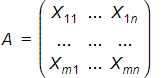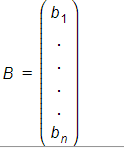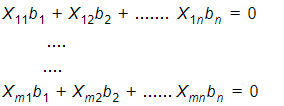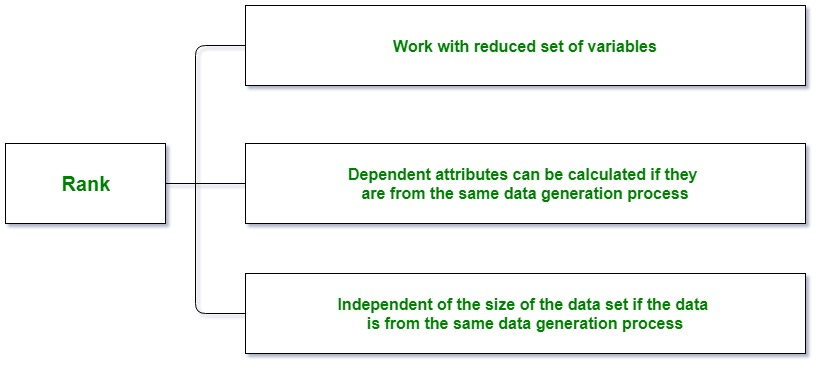Related Articles

# Null Space and Nullity of a Matrix

• Difficulty Level : Hard
• Last Updated : 14 May, 2019

Null Space and Nullity are concepts in linear algebra which are used to identify the linear relationship among attributes.

### Null Space:

The null space of any matrix A consists of all the vectors B such that AB = 0 and B is not zero. It can also be thought as the solution obtained from AB = 0 where A is known matrix of size m x n and B is matrix to be found of size n x k. The size of the null space of the matrix provides us with the number of linear relations among attributes.

Attention reader! Don’t stop learning now. Get hold of all the important Machine Learning Concepts with the Machine Learning Foundation Course at a student-friendly price and become industry ready.

A generalized description:

Let a matrix beand there is one vector in the null space of A, i.e,then B satisfies the given equations,The idea –

1. AB = 0 implies every row of A when multiplied by B goes to zero.
2. Variable values in each sample(represented by a row) behave the same.
3. This helps in identifying the linear relationships in the attributes.
4. Every null space vector corresponds to one linear relationship.

### Nullity:

Nullity can be defined as the number of vectors present in the null space of a given matrix. In other words, the dimension of the null space of the matrix A is called the nullity of A. The number of linear relations among the attributes is given by the size of the null space. The null space vectors B can be used to identify these linear relationship.

Rank Nullity Theorem:
The rank-nullity theorem helps us to relate the nullity of the data matrix to the rank and the number of attributes in the data. The rank-nullity theorem is given by –

Nullity of A + Rank of A = Total number of attributes of A (i.e. total number of columns in A)

Rank:
Rank of a matrix refers to the number of linearly independent rows or columns of the matrix.Example with proof of rank-nullity theorem:

Consider the matrix A with attributes {X1, X2, X3}
1  2  0
A = 2  4  0
3  6  1
then,
Number of columns in A = 3R1 and R3 are linearly independent.
The rank of the matrix A which is the
number of non-zero rows in its echelon form are 2.
we have,
AB = 0Then we get,
b1 + 2*b2 = 0
b3 = 0
The null vector we can get isThe number of parameter in the general solution is the dimension
of the null space (which is 1 in this example). Thus, the sum of
the rank and the nullity of A  is 2 + 1 which
is equal to the number of columns of A.


This rank and nullity relationship holds true for any matrix.

Python Example to find null space of a Matrix:

 # Sympy is a library in python for # symbolic Mathematicsfrom sympy import Matrix  # List A A = [[1, 2, 0], [2, 4, 0], [3, 6, 1]]  # Matrix AA = Matrix(A)  # Null Space of ANullSpace = A.nullspace()   # Here NullSpace is a list  NullSpace = Matrix(NullSpace)   # Here NullSpace is a Matrixprint("Null Space : ", NullSpace)  # checking whether NullSpace satisfies the# given condition or not as A * NullSpace = 0# if NullSpace is null space of Aprint(A * NullSpace)

Output:

Null Space :  Matrix([[-2], , ])
Matrix([, , ])


Python Example to find nullity of a Matrix:

 from sympy import Matrix  A = [[1, 2, 0], [2, 4, 0], [3, 6, 1]]   A = Matrix(A)  # Number of ColumnsNoC = A.shape  # Rank of Arank = A.rank()  # Nullity of the Matrixnullity = NoC - rank  print("Nullity : ", nullity)

Output:

Nullity :  1


My Personal Notes arrow_drop_up